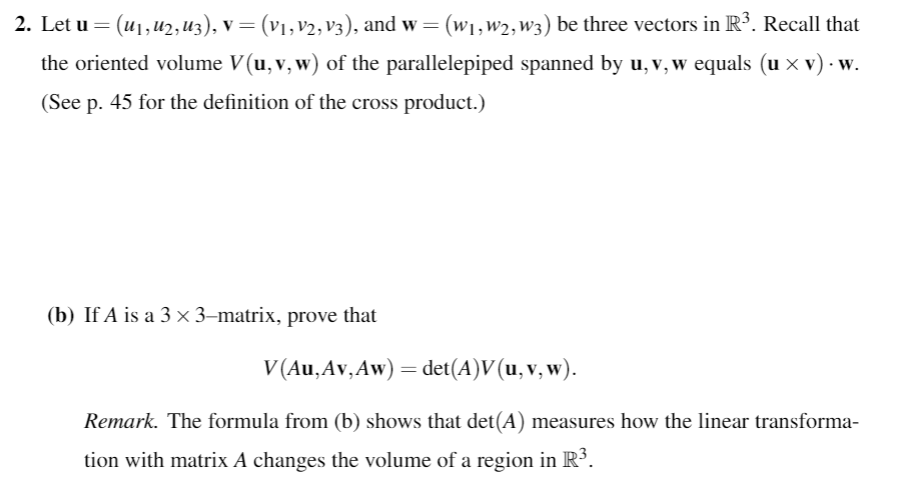# 2. Let u-("і "2,W3), ν(η , v 2, vs), and w-( w. w2,W3 ) be three vectors in R. Recall thatthe oriented volume V(u,v,w) of the parallelepiped spanned by u,v,w equals (их v) . w(See p. 45 for the definition of the cross product.)(b) If A is a 3 x 3-matrix, prove thatV(Au,Av,Aw)-det(A)V(u, v, w).Remark. The formula from (b) shows that det(A) measures how the linear transformation with matrix A changes the volume of a region in R5.

Question
8 viewshelp_outlineImage Transcriptionclose2. Let u-("і "2,W3), ν (η , v 2, vs), and w-( w. w2,W3 ) be three vectors in R. Recall that the oriented volume V(u,v,w) of the parallelepiped spanned by u,v,w equals (их v) . w (See p. 45 for the definition of the cross product.) (b) If A is a 3 x 3-matrix, prove that V(Au,Av,Aw)-det(A)V(u, v, w). Remark. The formula from (b) shows that det(A) measures how the linear transforma tion with matrix A changes the volume of a region in R5. fullscreen
check_circle

Step 1

Consider the matrix A as

Step 2

In order to show V(Au, Av, Aw)= det(A)V(u, v, w), prove that the left hand side is equal to right hand side of the equation.

Step 3

Compute the cross prod...

### Want to see the full answer?

See Solution

#### Want to see this answer and more?

Solutions are written by subject experts who are available 24/7. Questions are typically answered within 1 hour.*

See Solution
*Response times may vary by subject and question.
Tagged in

### Math